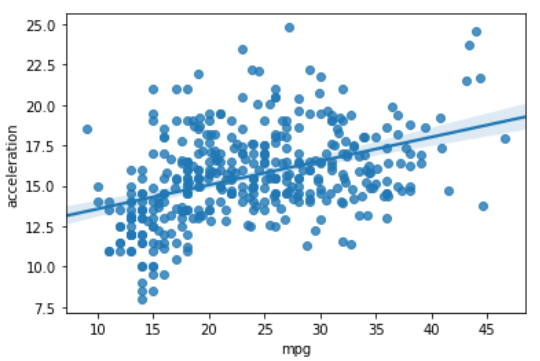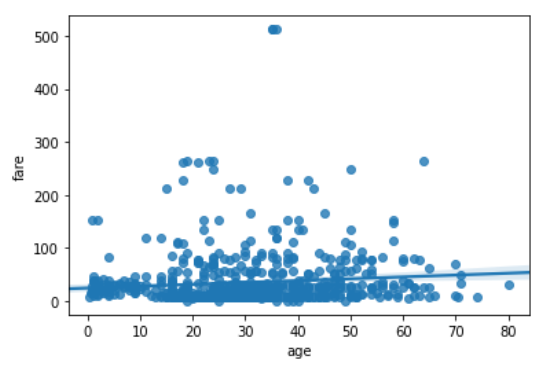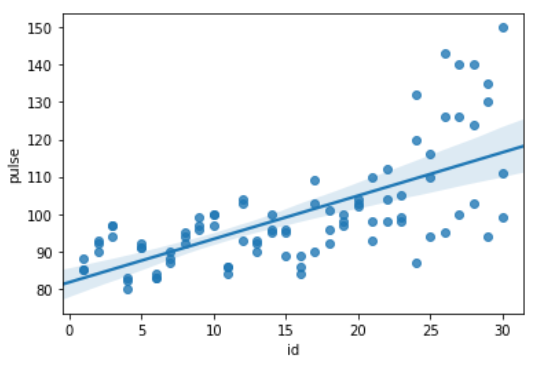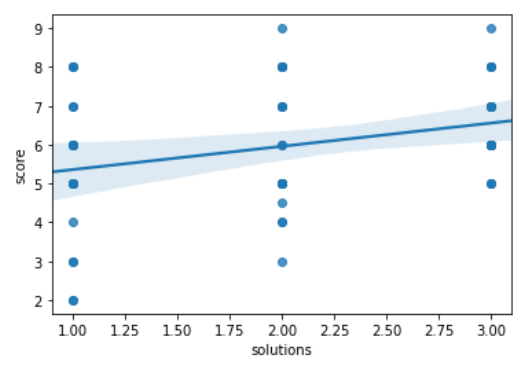# Python – seaborn.regplot() method

Seaborn is a Python data visualization library based on matplotlib. It provides a high-level interface for drawing attractive and informative statistical graphics. Seaborn helps resolve the two major problems faced by Matplotlib; the problems are ?

• Default Matplotlib parameters
• Working with data frames

As Seaborn compliments and extends Matplotlib, the learning curve is quite gradual. If you know Matplotlib, you are already half-way through Seaborn.

### seaborn.regplot() :

This method is used to plot data and a linear regression model fit. There are a number of mutually exclusive options for estimating the regression model. For more information click here.

Syntax : seaborn.regplot( x,  y,  data=None, x_estimator=None, x_bins=None,  x_ci=’ci’, scatter=True, fit_reg=True, ci=95, n_boot=1000, units=None, order=1, logistic=False, lowess=False, robust=False, logx=False, x_partial=None, y_partial=None, truncate=False, dropna=True, x_jitter=None, y_jitter=None, label=None, color=None, marker=’o’,    scatter_kws=None, line_kws=None, ax=None)

Parameters: The description of some main parameters are given below:

• x, y: These are Input variables. If strings, these should correspond with column names in “data”. When pandas objects are used, axes will be labeled with the series name.
• data:  This is dataframe where each column is a variable and each row is an observation.
• lowess: (optional) This parameter take boolean value. If “True”, use “statsmodels” to estimate a nonparametric lowess model (locally weighted linear regression).
• color: (optional) Color to apply to all plot elements.
• marker: (optional) Marker to use for the scatterplot glyphs.

Return: The Axes object containing the plot.

Below is the implementation of above method:

Example 1:

## Python3

 `# importing required packages ` `import` `seaborn as sns ` `import` `matplotlib.pyplot as plt ` ` `  `# loading dataset ` `data ``=` `sns.load_dataset(``"mpg"``) ` ` `  `# draw regplot ` `sns.regplot(x ``=` `"mpg"``,  ` `            ``y ``=` `"acceleration"``,  ` `            ``data ``=` `data) ` ` `  `# show the plot ` `plt.show() ` ` `  `# This code is contributed  ` `# by Deepanshu Rustagi.`

Output :Example 2:

## Python3

 `# importing required packages ` `import` `seaborn as sns ` `import` `matplotlib.pyplot as plt ` ` `  `# loading dataset ` `data ``=` `sns.load_dataset(``"titanic"``) ` ` `  `# draw regplot ` `sns.regplot(x ``=` `"age"``, ` `            ``y ``=` `"fare"``, ` `            ``data ``=` `data, ` `            ``dropna ``=` `True``) ` `# show the plot ` `plt.show() ` ` `  `# This code is contributed  ` `# by Deepanshu Rustagi.`

Output :Example 3:

## Python3

 `# importing required packages ` `import` `seaborn as sns ` `import` `matplotlib.pyplot as plt ` ` `  `# loading dataset ` `data ``=` `sns.load_dataset(``"exercise"``) ` ` `  `# draw regplot ` `sns.regplot(x ``=` `"id"``, ` `            ``y ``=` `"pulse"``,  ` `            ``data ``=` `data) ` ` `  `# show the plot ` `plt.show() ` ` `  `# This code is contributed  ` `# by Deepanshu Rustagi.`

Output :Example 4 :

## Python3

 `# importing required packages ` `import` `seaborn as sns ` `import` `matplotlib.pyplot as plt ` ` `  `# loading dataset ` `data ``=` `sns.load_dataset(``"attention"``) ` ` `  `# draw regplot ` `sns.regplot(x ``=` `"solutions"``, ` `            ``y ``=` `"score"``, ` `            ``data ``=` `data) ` ` `  `# show ther plot ` `plt.show() ` ` `  `# This code is contributed  ` `# by Deepanshu Rustagi.`

Output :My Personal Notes arrow_drop_upCheck out this Author's contributed articles.

If you like GeeksforGeeks and would like to contribute, you can also write an article using contribute.geeksforgeeks.org or mail your article to contribute@geeksforgeeks.org. See your article appearing on the GeeksforGeeks main page and help other Geeks.

Please Improve this article if you find anything incorrect by clicking on the "Improve Article" button below.

Article Tags :

Be the First to upvote.

Please write to us at contribute@geeksforgeeks.org to report any issue with the above content.